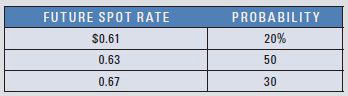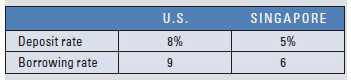# Comparison of Techniques for Hedging Receivables Assume that Carbondale Co. expects to receive \$500,000 in one year. The existing spot rate of the singapore dollar is \$0.60. The one-year for ward rate of the singapore dollar is \$0.62. Carbondale created the following probability distribution for the future spot rate in one year: Assume that one-year put options on singapore dollars are available, with an exercise price of \$0.63 and a premium of \$0.04 per unit. One-year call options on singapore dollars are available with an exercise price of \$0.60 and a premium of \$0.03 per unit. Assume the following money market rates: Given this information, determine whether a forward hedge, a money market hedge, or a currency options hedge would be most appropriate. Then compare the most appropriate hedge to an unhedged strategy, and decide whether Carbondale should hedge its receivables position. Assume that Baton Rouge, Inc., expects to need \$1 million in one year. Using any relevant information in part (a) of this question, determine whether a for ward hedge, a money market hedge, or a currency options hedge would be most appropriate. Then compare the most appropriate hedge to an unhedged strategy, and decide whether Baton Rouge should hedge its payables position.FindFind

### International Financial Management

14th Edition
Madura
Publisher: Cengage
ISBN: 9780357130698FindFind

### International Financial Management

14th Edition
Madura
Publisher: Cengage
ISBN: 9780357130698

#### Solutions

Chapter 11, Problem 32QA
Textbook Problem

## Comparison of Techniques for Hedging Receivables Assume that Carbondale Co. expects to receive \$500,000 in one year. The existing spot rate of the singapore dollar is \$0.60. The one-year for ward rate of the singapore dollar is \$0.62. Carbondale created the following probability distribution for the future spot rate in one year:Assume that one-year put options on singapore dollars are available, with an exercise price of \$0.63 and a premium of \$0.04 per unit. One-year call options on singapore dollars are available with an exercise price of \$0.60 and a premium of \$0.03 per unit. Assume the following money market rates:Given this information, determine whether a forward hedge, a money market hedge, or a currency options hedge would be most appropriate. Then compare the most appropriate hedge to an unhedged strategy, and decide whether Carbondale should hedge its receivables position. Assume that Baton Rouge, Inc., expects to need \$1 million in one year. Using any relevant information in part (a) of this question, determine whether a for ward hedge, a money market hedge, or a currency options hedge would be most appropriate. Then compare the most appropriate hedge to an unhedged strategy, and decide whether Baton Rouge should hedge its payables position.

This textbook solution is under construction.

## Expert Solution

### Want to see the full answer?

Check out a sample textbook solution.

### Want to see this answer and more?

Experts are waiting 24/7 to provide step-by-step solutions in as fast as 30 minutes!*

*Response times vary by subject and question complexity. Median response time is 34 minutes and may be longer for new subjects.© 2021 bartleby. All Rights Reserved.